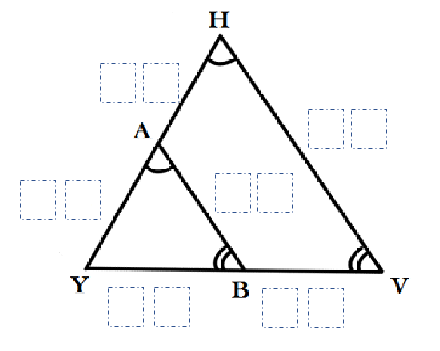# Similar Triangles 2

Directions: Using the digits 0 to 9 at most one time each, create two similar triangles. You may have as many leading zeros as you like.### Hint

Can you use a scale factor to help you in any way?
What is true about the corresponding sides of similar triangles?

There are many answers. Here are the possibilities:
YA – 5
AH – 10
YB – 3
BV – 6
BA – 9
VH – 27

Source: Drew Ross

## Three Triangles And A Wannabe

Directions: Using the values 8, 10, 12, 14, 16, 18, and 20, determine lengths for …

1.In your solution the sides of your smaller triangle are 3, 5, and 9. There is no such possible triangle as the sun of any two sides of a triangle must be greater than the third. 3 + 5 = 8, thus you cannot have a 3,5,9 triangle. The larger triangle is also impossible since it is a dilation of the smaller figure.

2.Here’s one solution I found…

YA – 4
AH – 8
YB – 5
BV – 10
BA – 3
VH – 9